## Section2.4Velocity and Speed

### Subsection2.4.1Average Speed

Perhaps the crudest measure of how fast something is moving comes from looking at the distance it has covered in a given time. The ratio of the distance convered, $d \text{,}$ and the time taken, $t \text{,}$ is called average speed. We will denote average speed by the symbol $v_{\text{s,av}}\text{,}$ and if there is no confusion in the context, then by simply $v\text{.}$

\begin{equation} v_{\text{s,av}} = \dfrac{d}{t}.\tag{2.4.1} \end{equation}

Suppose you drive a car for a distance of $4000\text{ km}$ in $50\text{ hr}\text{.}$ Then your average speed will be

\begin{equation*} v_{\text{s,av}} = \dfrac{4000\text{ km}}{50\text{ hr}} = 80\text{ km/hr}. \end{equation*}

Note that when we are speaking about average speed, we are not concerned with the direction changes during the motion or the overall direction of motion. These will be important for velocity, not for speed.

You drive your car from New York City to Los Algeles in 50 h at an average speed of 96 km/h. What is the total distance you have traveled?

Hint

Use the definition of average speed.

$4,800 \text{ km}$ .

Solution
From the definition of average speed, $v = d/t \text{,}$ we get
\begin{equation*} d = v t = 96 \text{ km/h } \times 50 \text{ h } = 4,800 \text{ km}. \end{equation*}

### Subsection2.4.2Average Velocity

Another measure of motion has to with the rate of overall movement from the intitial instant to the final instant. Here, we do not care about all the places you visited during the interval, but just where you were at the beginning and where you ended up at the end, i.e., we care about displacement during the interval and not the distance covered. Average velocity is obtained by dividing the displacment by the interval.

If the motion is on the $x$ axis, we will denote the average velocity by the symbol $v_{\text{av},x}\text{,}$ which will be the ratio of the displacement, $\Delta x \text{,}$ and the time interval, $\Delta t \text{.}$

\begin{equation} v_{\text{av},x} = \dfrac{\Delta x}{\Delta t}.\tag{2.4.2} \end{equation}

Suppose you go from one end A of the room to another end B, say $3\text{ m}$ from A, and then track back $1\text{ m}$ to C.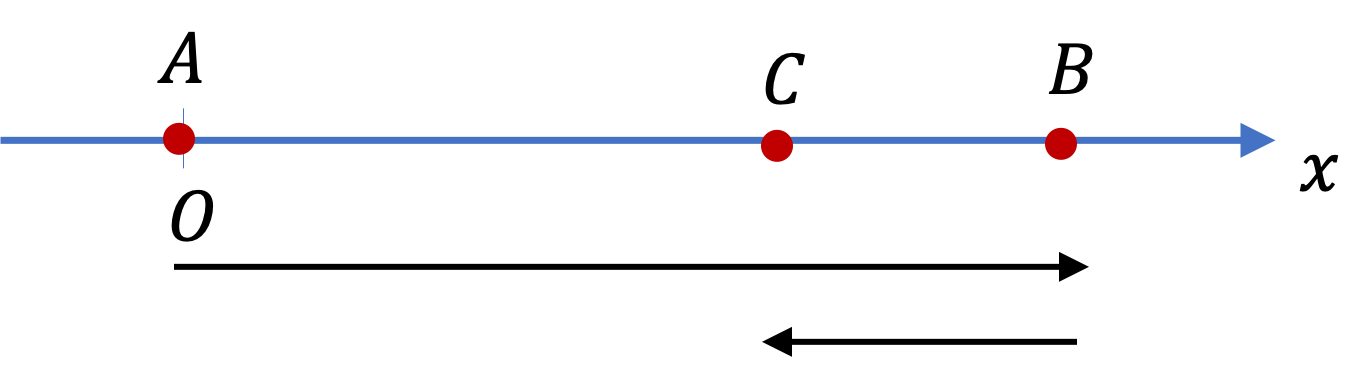Suppose you use positive $x$ axis in the direction A-to-B. You would have covered a distance

\begin{equation*} d = 3 + 1 = 4\text{ m}, \end{equation*}

\begin{equation*} \Delta x = 2\text{ m}. \end{equation*}

The difference is due to the fact that tracking back canceled out part of the original displacement and you ended up at $x = 2\text{ m}$ from the starting place A. Now, say A-to-B-to-C took $5\text{ sec}\text{,}$ then your average velocity and average speed will be

\begin{align*} \amp v_{\text{av},x} = \dfrac{2}{5} = 0.4\text{ m/s},\\ \amp v_{s,\text{av}} = \dfrac{4}{5} = 0.8\text{ m/s}. \end{align*}

Since we used $x$ axis for the displacement, we refer to this average velocity as average $x$ velocity. If we had used $y$ axis along ACB, we would have called it average $y$ velocity. After we have studied vectors, you will find out that velocity is a vector quantity, while speed is not a vector.

Average $x$ velocity will be negative if overall motion from start to finish is facing towards $x=-\infty\text{.}$

For instance, say you move from A-to-B-to-C in $2\text{ sec}\text{.}$ Then, your verage $x$ velocity will be

\begin{equation*} v_{\text{av},x} = \dfrac{1\text{ m} - 4\text{ m}}{2\text{ s}} = -1.5\text{ m/s}. \end{equation*}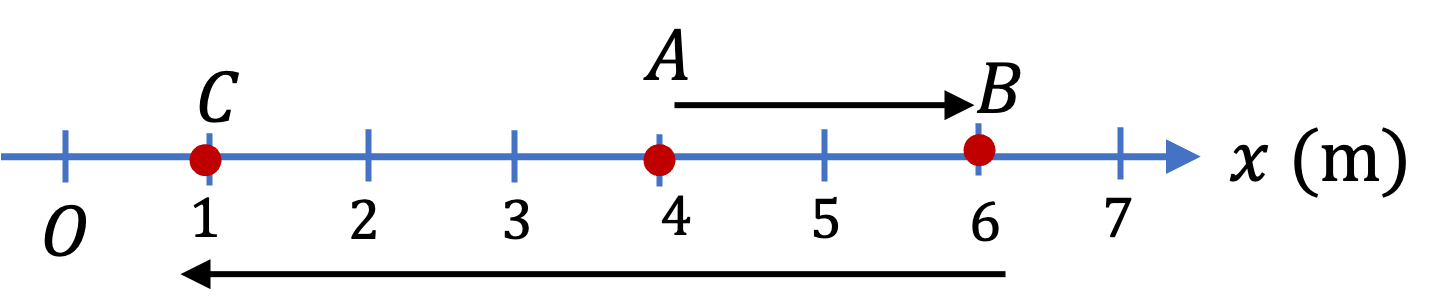Figure 2.4.2. A-to-C direction towards $x=-\infty\text{.}$
###### Remark2.4.3.

If there was no turn back in the motion, as in the A-to-B part of your motion, we would get the same absolute values for the displacement and the distance traveled. In this case, average speed will equal to the absolute value of the average velocity.

Now, notice an amazing fact. If the interval of interest is an infinitesimal interval of time, meaning as small an interval as you like, except zero interval. In such an interval, since there will be no turn around, the magnitude of average speed and average velocity will be same. In this case, we call them instantaneous speed and instantaneous velocity.

A ball is rolling on a straight track. At the end of the track, the ball hits a wall and bounces back with the same speed. Ignore the time the ball was in contact with the wall during the collision. Let the original direction of ball be the positive $x$ axis direction.

Let the ball be at $x = 15\text{ cm}$ at $t = 0 \text{,}$ and at $x = 75\text{ cm}$ at $t = 10\text{ sec} \text{.}$ The wall is at $x = 100\text{ cm}\text{.}$ Suppose the collision occured sometime between $t=0$ and $t=10\text{ sec}\text{.}$

What are (a) the average velocity and (b) average speed during the $t = 0$ to $t = 10\text{ sec}$ interval?

Hint

Average velocity on $x$ axis is based on $x$ coordinates and average speed is based on actual distance covered.

(a) $6 \text{ cm/s}\text{,}$ (b) $11 \text{ cm/s}\text{.}$

Solution

(a) Average Velocity :

The definition of average velocity for a one-dimensional motion on $x$ axis is

\begin{equation*} v_{\text{av},x} = \dfrac{\Delta x }{\Delta t}. \end{equation*}

Therefore,

\begin{equation*} v_{\text{av},x} = \dfrac{75\text{ cm} - 15\text{ cm}}{10\text{ sec}} = 6 \text{ cm/s}. \end{equation*}

(a) Average Speed

The definition of average speed for any motion is

\begin{equation*} v_{\text{s,av}} = \dfrac{d }{\Delta t}. \end{equation*}

The motion has two legs here: (1) from $x = 15\text{ cm}$ to $x = 100\text{ cm}$ (i.e., the wall) and (2) from $x = 100\text{ cm}$ to $x = 75\text{ cm} \text{.}$ We need to add these distances, not just difference in coordinates. Therefore,

\begin{equation*} v_{\text{s,av}} = \dfrac{85\text{ cm} + 25\text{ cm}}{10\text{ sec}} = 11 \text{ cm/s}. \end{equation*}

### Subsection2.4.3Instantaneous Velocity

Often we want velocity at a particular instant, called instantaneous velocity, or simply, velocity. This refers to the average velocity between that instant, say $t \text{,}$ and an instant very close to $t\text{,}$ e.g., a tiny amount of time later than $t\text{,}$ which we can indicate by $t + \Delta t \text{.}$ Or, alternately, between $t$ and a tiny instant before $t\text{,}$ say at $t-\Delta t\text{.}$

How close instants $t$ and $t + \Delta t$ should be? We say that we want them to be as close as possible except zero interval. You might complain that this is not good enough and that the answer is a little vague. You may already be familiar with it from your Calculus class since it is at the heart of the concept of taking the limit. We call this notion of interval an infintesimal interval. It is one of the most important concepts of physics.

Denoting instantaneous velocity for a motion along $x$ axis by symbol $v_x \text{,}$ we write this special averaging formally using the notation of taking a limit in Calculus,

\begin{equation} v_x = \lim_{\Delta t \rightarrow 0} \dfrac{x\left(\text{at } t+\Delta t\right) - x\left(\text{at } t\right)}{\Delta t}.\label{eq-instantaneous-velocity-1d-limit}\tag{2.4.3} \end{equation}

This is also denoted by the derivative symbol.

\begin{equation} v_x = \dfrac{dx}{dt}.\tag{2.4.4} \end{equation}

which is also denoted by the derivative symbol $dx/dt\text{.}$ Since derivative of a function has graphical interpretation of slope, we can compute $v_x$ from the slope of $x$ versus $t$ plot. We will see that below.

When the object is moving towards $x = +\infty\text{,}$ we will have $x\left(\text{at } t+\Delta t\right) \gt x\left(\text{at } t\right)\text{,}$ which will give positive $v_x\text{.}$ And, when the object is moving towards $x = -\infty\text{,}$ we will have $x\left(\text{at } t+\Delta t\right) \lt x\left(\text{at } t\right)\text{,}$ which will give negative $v_x\text{.}$

The requirement that $t+\Delta t$ be as close to $t$ as possible makes the turn around in motion between $t$ and $t+\Delta t$ impossible. That means, the magnitude of instantaneous velocity, i.e., the value without the sign, will correspond to speed at that instant, i.e., instantaneous speed. We will denote instantaneous speed by symbol $v$ without $x\text{.}$

\begin{equation} v = |v_x|.\tag{2.4.5} \end{equation}

Note that while $v_x$ can be positive or negative, $v$ will always be positive. Here, we have worked with motion that falls along only the $x$ axis. We will find in a later chapter that, a general motion can be thought as “motion along $x\text{,}$ $y\text{,}$ and $z$ coordinates” with velocity (components) $v_x\text{,}$ $v_y\text{,}$ and $v_z$ along them. The speed is then related to the adding these velocities in quadrature.

\begin{equation} v = \sqrt{v_x^2 + v_y^2 + v_z^2}. \tag{2.4.6} \end{equation}

### Subsection2.4.4Graphical Definition of Velocity

Instantaneous velocity for a motion along one axis can also be defined graphically in addition to its anlaytic definition using the limit concept of Calculus decribed above.

Figure Figure 2.4.6 shows an $x$ versus $t$ plot of a motion that occurs on $x$ axis. Since the plot has two linear segments, there will be two velociy values.

\begin{align*} \amp v_x = 300/20 = 15\text{ m/s} \ \ ( 0\lt t \lt 20\text{ sec} )\\ \amp v_x = -400/50 = -8\text{ m/s} \ \ ( 20\text{ sec}\lt t \lt 70\text{ sec}) \end{align*}

The velocity $v_x$ in the second segment is negative since the object is moving towards negative $x$ axis. Clearly, in the linear segments, i.e., where the velocity is steady, velocity $v_x$ is simply slope of $x$ vs $t$ plot.

\begin{equation*} v_x = \text{ slope of }x \text{ versus }t. \end{equation*}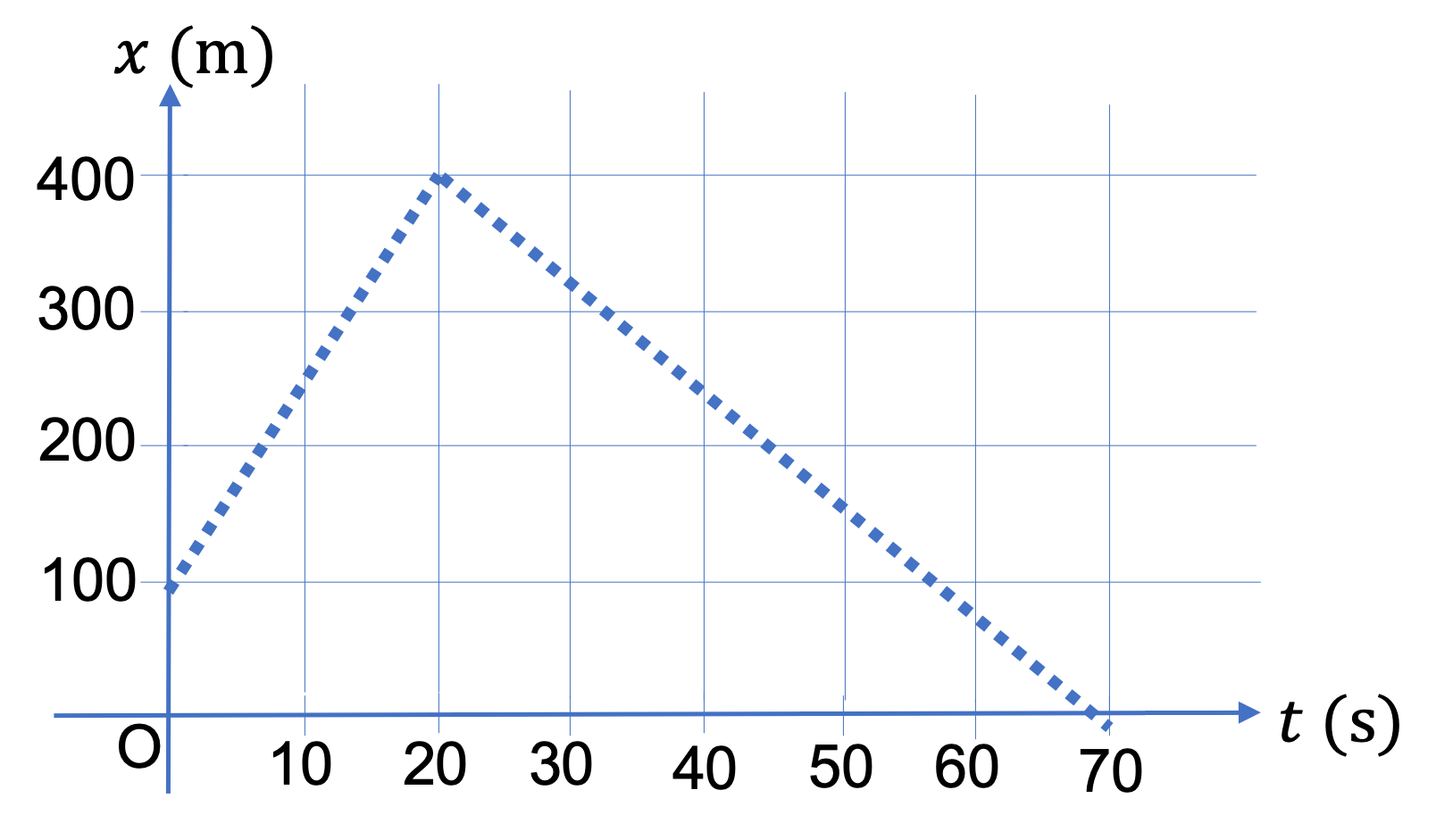Figure 2.4.6. The slope of $x$ versus $t$ plot gives the velocity $v_x \text{.}$

What about the times when the velocity is changing, e.g., at $t=20\text{ sec}$ in the figure? Derivative does not exist at this point, and hence, we cannot get any value of velocity. But, physical velocity of an object is well-defined at al times. So, what's the problem here? It turns out that figures like these are wrong - in reality, you will get a plot that is smooth at the tip, not pointed as shown. this is just an artifact of this type of drawing which is common in early textbooks.

For another example, consider plot of $x$ verus $t$ given in Figure 2.4.7. The object is moving steadily between B and C. Thefore, in this segment, the plot is a straight line. From the slope of this line we can get $v_x$ between B and C.

The motion is not steady between A and B, since the object is slowing down, or or between C and D, where it is speeding up. The plot of $x$ versus $t$ is curved during them, which means there is not one slope. The slope now refers to the slope of tangent lines. Therefore, we now need to draw tangent lines at each instant and compute their slopes.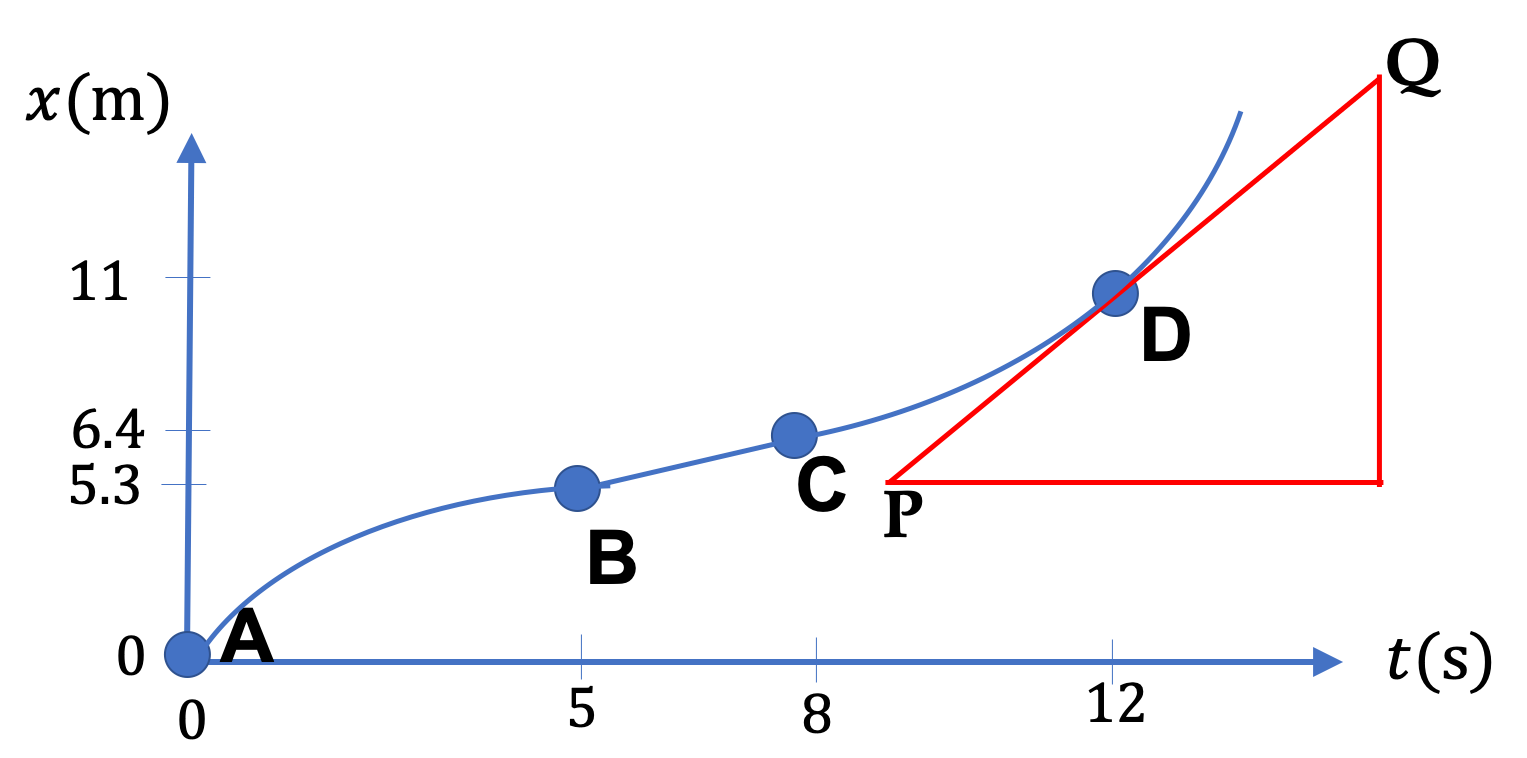Figure 2.4.7. Figure 2.2.3 is reproduced here to illustrate the computation of velocity by slope of tangent line to the $x$ versus $t$ plot.

Suppose we want velocity at D. We will draw a tangent to the curve at this point, e.g., line PQ that touches the curve at the point of interest. Then, we compute instantaneous velocity $v_x$ at D from the slope of tangent PQ.

You can see that between A and B, the absolute value of slopes of tangent lines are getting smaller - hence motion is slowing down. The slopes at various instants between C and D will convince you that their slopes are getting larger, hence the motion is speeding up.

The $x$ coordinate of a runner plotted against time $t$ as in the figure below.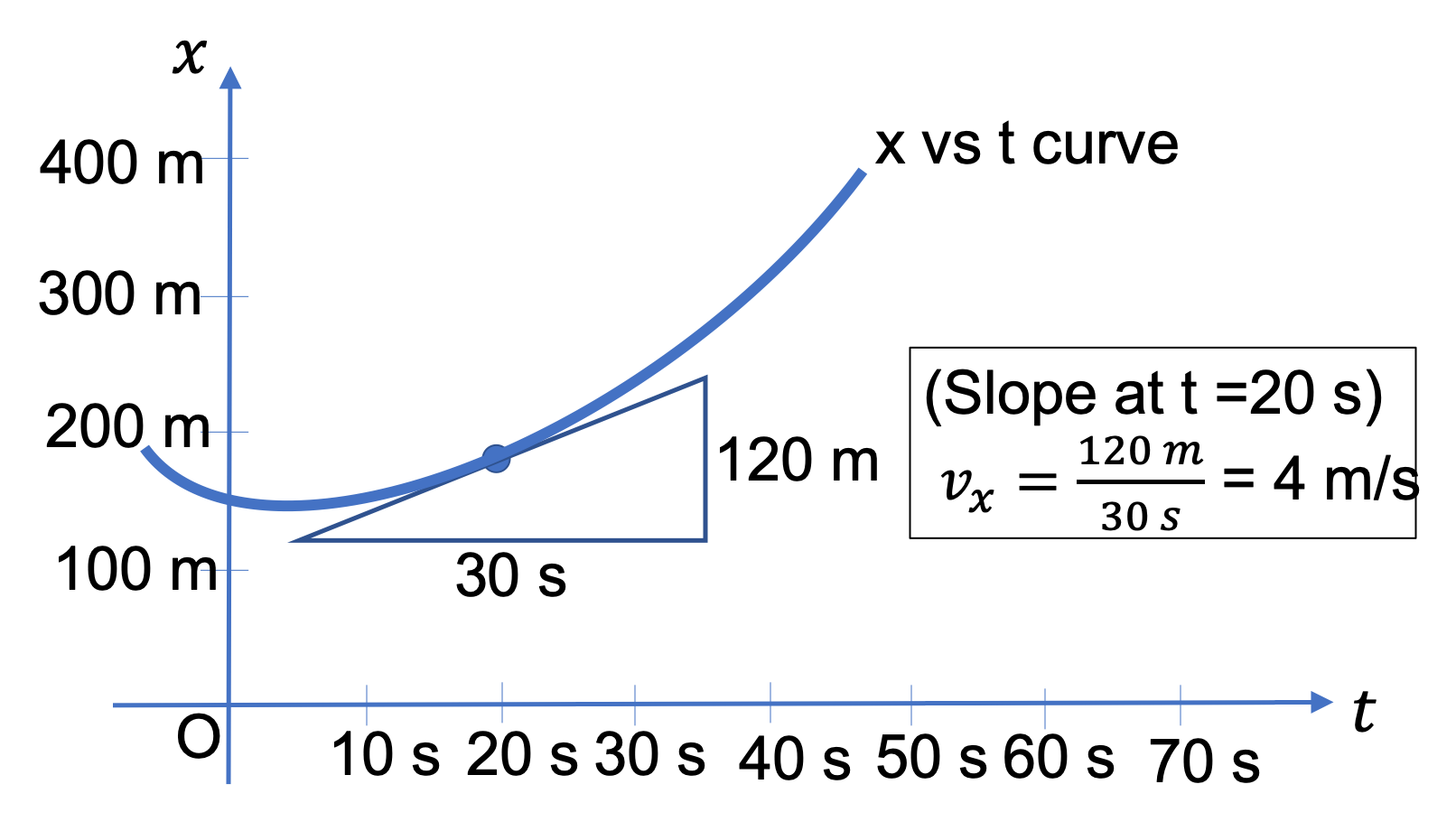We draw a tangent to the $x$ versus $t$ graph at the instant of interest, here $t = 20$ sec. The slope of the tangent line gives the numerical value of the $x$-velocity. Here $x$-velocity is positive since the runner's $x$ coordinate is increasing with time. That is, velocity has magnitude $4.0$ m/s in the positive $x$ direction.

You can see that the slope of the tangent has different values at different instants. Try looking at the slope at other times. This is a situation in which velocity $v_x$ is changing with time. Can you find an instant when the $x$-velocity is zero in the figure? Hint: Look for a place where the tangent line is horizontal.

A train is moving on a straight track. Taking the track to be the $x$ axis, the position of the train was noted at various times. The data was then plotted with $t$ along the abscissa and $x$ along the ordinate as shown in Figure 2.4.10. What are the values of $v_x$ at the following instants (a) $t = 0$ sec, (b) $t = 10$ sec, (c) $t = 25$ sec, (d) $t = 35$ sec, (e) $t = 50$ sec, and (f) $t = 70$ sec?

Hint

Read off two nearby points on the graph and compute the slope.

(a) 5.0 m/s, (b) 5.0 m/s, (c) 20.0 m/s, (d) 0, (e) $- 15.0$ /s, (f) 0.
Solution

(a) Note that at $t = 0 \text{,}$ the slope is not zero if we assume that the line contnues past $t = 0$ to the negative time. To determine $v_x$ here, you read off two nearby points on the graph. For $t = 0 \text{,}$ we will use $(t_1,x_1) = (0, 0)$ and $(t_2,x_2) = (20\text{ s}, 100\text{ m}) \text{.}$ From these we get $v_x$ at $t=0 \text{.}$

\begin{equation*} v_x = \dfrac{x_2-x_1}{t_2-t_1} = \dfrac{100\text{ m} - 0}{20\text{ s} - 0} = 5.0\, \frac{\text{m}}{\text{s}}. \end{equation*}

(b) For $t = 10$ sec, we take points at $t = 0$ and $t = 20$ sec. We get the same value as in (a).

(c) For $t = 25$ sec, we take points at $t = 20$ sec and $t = 30$ sec, viz., $(t_1,x_1) = (20 \text{ s}, 100 \text{ m})$ and $(t_2,x_2) = (30\text{ s}, 300 \text{ m}) \text{.}$ From these we get $v_x$ at $t = 25$ sec.

\begin{equation*} v_x = \dfrac{x_2-x_1}{t_2-t_1} = \dfrac{300\text{ m} - 100\text{ m}}{30\text{ s} - 20\text{ s}} = 20.0\, \frac{\text{m}}{\text{s}}. \end{equation*}

(d) There is no change in $x$ around this time point. So, $v_x = 0$ at $t = 35$ sec.

(e) For $t = 50$ sec, we take points at $t = 40$ sec and $t = 60$ sec, viz., $(t_1,x_1) = (40 \text{ s}, 300 \text{ m})$ and $(t_2,x_2) = (60\text{ s}, 0 ) \text{.}$ From these we get $v_x$ at $t = 50$ sec.

\begin{equation*} v_x = \dfrac{x_2-t_2}{x_1-t_1} = \dfrac{0\text{ m} - 300\text{ m}}{60\text{ s} - 40\text{ s}} = -15.0\, \frac{\text{m}}{\text{s}}. \end{equation*}

Note that $v_x$ here is negative. This is due to the fact that the train is moving in the same direction as the negative $x$ axis.

(f) There is no change in $x$ around this time point. Therefore, $v_x = 0$ here.

### Subsection2.4.5Displacement from Velocity Graphically

We saw above that we can get velocity along $x$ axis from the slope of tangent to the plot of $x$ versus $t \text{,}$ in place of computing derivative of the function $x(t) \text{.}$

Now, we want to ask the inverse question: how can we get $\Delta x$ if we have a plot of $v_x$ versus $t\text{?}$ Fundamental theorem of Calculus already addresses this], i.e., “integral is inverse of derivative”. So, $\Delta x$ will be obtained by integrating $v_x\text{.}$

Anayltically, an integral can be thought of as a sum, which is related to “area under the curve” in a graph. Here, we will be interested in area between the $v_x$ plot curve and the $v_x = 0$ line - if $v_x \gt 0\text{,}$ we will get positive area and if $v_x \lt 0\text{,}$ we will get negative area. Calculation of displacement $\Delta x$ from a $v_x$ versus $t$ plot is illustrated in Figure 2.4.11.

\begin{equation} \Delta x \equiv x(t_f) - x(t_i) = \text{Area under the }v_x\text{ verus } t\text{ plot.}\label{eq-1d-review-displacement-from-vx-graphical}\tag{2.4.7} \end{equation}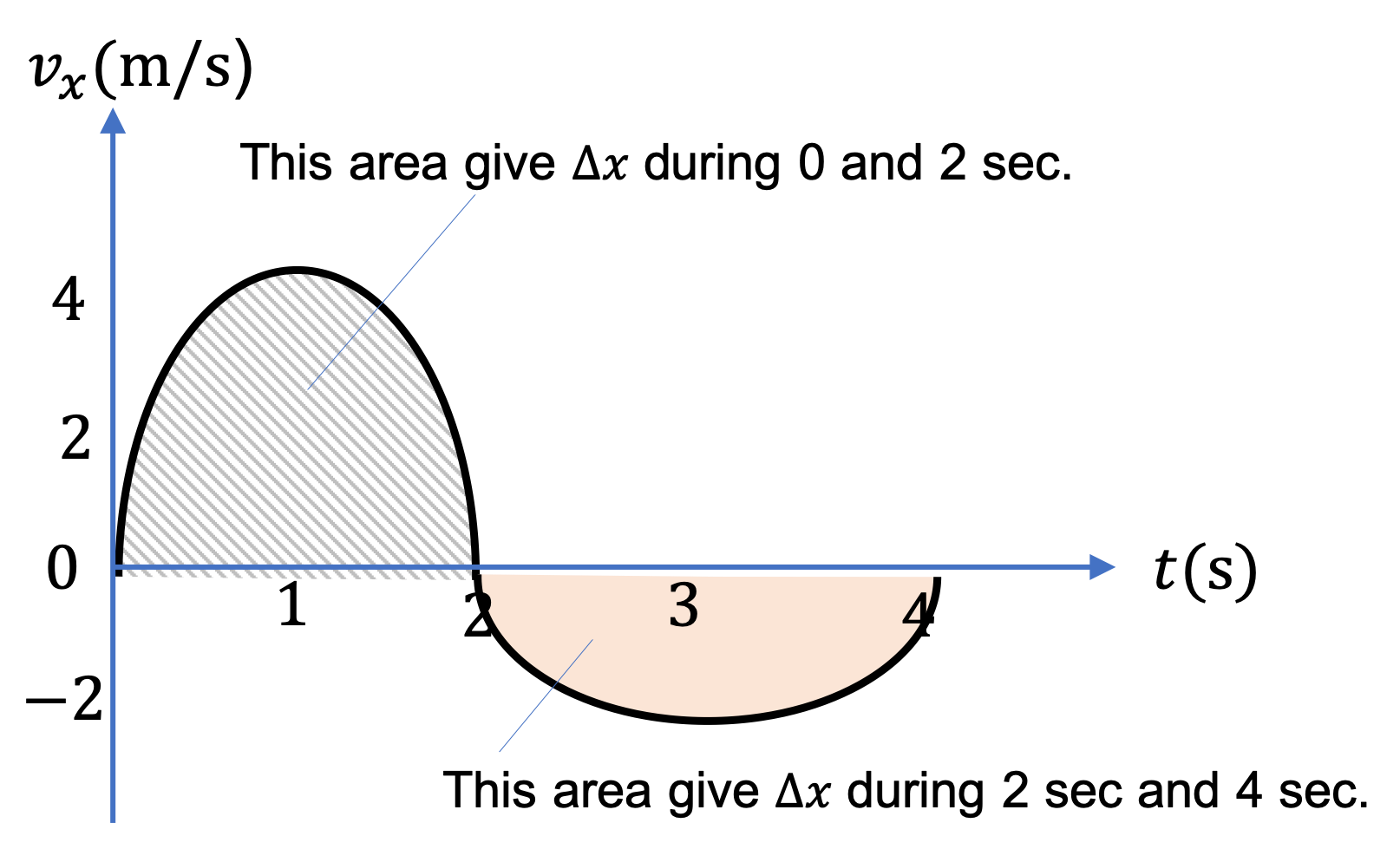Figure 2.4.11. The area under the curve to get $\Delta x$ from $v_x$ versus $t$ plot. The area for the curve when $v_x \gt 0$ is positive, as between $t=0$ and $t= 2\text{ sec}$ and the area for the curve when $v_x \lt 0$ is negative, as between $t= 2\text{ sec}$ and $t= 4\text{ sec}\text{.}$

The instantaneous $v_x$ of a car moving on a straight road is plotted against time $t$ as shown in Figure 2.4.13. Find the displacements of $x$ coordinate of the car during the following intervals of time:

(a) $t = 0 \rightarrow 20 \text{ s}\text{,}$ (b) $t = 20 \text{ s}\rightarrow 40 \text{ s}\text{,}$ and (c) $t = 40 \text{ s}\rightarrow 70 \text{ s}\text{.}$

Note that area can be negative here corresponding to $x_f - x_i \lt 0 \text{.}$

Hint

(a) The area here is the area of a traingle. (b) The positive and negative areas cancel. (c) The area here is negative.

(a) $200\test{ m}\text{,}$ (b) 0, (c) $-600\text{ m}\text{.}$

Solution 1 (a)

(a) We wish to find the area under the curve (which is a straight line here) between $t = 0$ and $t = 20 \text{ s}$ - the shaded area in Figure 2.4.14. This is just the area of the triangle with base = 20 sec, and height = 20 m. Therefore,

\begin{equation*} x_f - x_i = \dfrac{1}{2} \times 20 \text{ m/s} \times 20 \text{ s} = 200 \text{ m}. \end{equation*}
Solution 2 (b)

(b) We wish to find the area under the curve between $t = 20 \text{ s}$ and $t = 40 \text{ s}$ in Figure 2.4.15. This is sum of areas of two triangles, a triangle above the time axis (where $v_x \gt 0$) and another below the time axis (where $v_x \lt 0$). The area of the triangle with $v_x \gt 0$ will give positive area and the other one will give negative area. They happen to cancel out.

\begin{equation*} x_f - x_i = \dfrac{1}{2} \times 20 \text{ m/s} \times 10 \text{ s} + \dfrac{1}{2} \times (-20) \text{ m/s} \times 10 \text{ s} = 0\text{ m}. \end{equation*}

This means that the car went 100 m towards positive $x$ axis and then backtracked same amount, ending up at the same $x$ at the end as at the beginning.

Solution 3 (c)

(c) We wish to find the area under the curve between $t = 40 \text{ s}$ and $t = 70 \text{ s}$ as shown in Figure 2.4.16. This is actually the area “above” the curve since the area of interest is the area between the curve and the abscissa of the plot.

\begin{equation*} x_f - x_i = \times (-20) \text{ m/s} \times 30 \text{ s} = -600\text{ m}. \end{equation*}

From the $v_x$ versus $t$ plot in Figure 2.4.18, find change in $x$ during the following intervals given in seconds (a) $(0, 2) \text{,}$ (b) $(2, 3) \text{,}$ (c) $(3, 4) \text{,}$ and (d) $(0, 4) \text{.}$

Hint

Use areas.

(a) $8\text{ m}\text{,}$ (b) $2\text{ m}\text{,}$ (c) $-1\text{ m}\text{,}$ (d) $9\text{ m}\text{.}$

Solution

(a) This is a rectangular area, of height $4\text{ m/s}$ and width $2\text{ sec}\text{.}$ The line is above the $v_x = 0$ line. Therefore, the change in $x$ will be:

\begin{equation*} \Delta x = 4\text{ m/s} \times 2\text{ s} = 8\text{ m}. \end{equation*}

(b) This is triangular area, of height $4\text{ m/s}$ and base $1\text{ sec}\text{.}$ The line is above the $v_x = 0$ line. Therefore, the change in $x$ will be:

\begin{equation*} \Delta x = \dfrac{1}{2}\times 4\text{ m/s} \times 1\text{ s} = 2\text{ m}. \end{equation*}

(c) This is triangular area, of height $2\text{ m/s}$ and base $1\text{ sec}\text{.}$ The line is below the $v_x = 0$ line. Therefore, the change in $x$ will be:

\begin{equation*} \Delta x = -\dfrac{1}{2}\times 2\text{ m/s} \times 1\text{ s} = -1\text{ m}. \end{equation*}

(d) This is just the sum of all the areas in (a), (b), and (c).

\begin{equation*} \Delta x = 8\text{ m} + 2\text{ m} -1\text{ m} = 9\text{ m}. \end{equation*}

The $v_x$ of a car moving on a straight road is plotted against time $t$ as shown in Figure 2.4.20. Find the displacements of the car during the following intervals of time: (a) $t = 0 \rightarrow 20 \text{ s}\text{,}$ (b) $t = 20 \text{ s}\rightarrow 40 \text{ s}\text{,}$ (c) $t = 40 \text{ s}\rightarrow 50 \text{ s}\text{,}$ (d) $t = 50 \text{ s}\rightarrow 70 \text{ s}\text{,}$ and (e) $t = 10 \text{ s}\rightarrow 20 \text{ s}\text{.}$

Hint

Find the areas between the plot line and the time axis.

(a) $200$ m, (b) $200$ m, (c) $-50$ m, (d) $-200$ m, (e) $150$ m.

Solution

The area under the plot (or, over the plot if $v_x \lt 0$) is the displacement. We use area of triangle = $\dfrac{1}{2} \times$ base $\times$ height and area of a rectangle = base $\times$ height.

(a) $\Delta x = 0.5 \times 20 \text{ s} \times 20 \text{ m/s} = 200 \text{ m}.$

(b) $\Delta x = 0.5 \times 20 \text{ s} \times 20 \text{ m/s} = 200 \text{ m}.$

(c) $\Delta x = 0.5 \times 10 \text{ s} \times (-10 \text{ m/s} ) = -50 \text{ m}.$

(d) $\Delta x = 20 \text{ s} \times (-10 \text{ m/s} ) = -200 \text{ m}.$

(e) This one is different - here we need to add two parts, one a triangle and the other a square.

$\Delta x = 0.5 \times 10 \text{ s} \times 10 \text{ m/s} + 10 \text{ s} \times (10 \text{ m/s} ) = 150 \text{ m}.$

### Subsection2.4.6(Calculus) Analytic Definition of Velocity

The fundamental definition of $v_x \text{,}$ the rate of change of $x$ is based on the observation in Calculus that derivative of a function is equal to the slope of the tangent line. Therefore, we define $v_x$ as derivative of $x$ versus $t \text{.}$

\begin{equation} v_x = \dfrac{dx}{dt}.\label{eq-velocity-1d-definition}\tag{2.4.8} \end{equation}

Note that $v_x$ can also change with time, and hence is also a function of $t \text{.}$ When we study the rate of change of $v_x$ we will get acceleration of the motion.

A block of copper attached to a spring executes a one-dimensional motion. The position of the center of the block varies with time according to the following function, $x(t) = 5.0\ \cos(2\pi t)\text{,}$ where $t$ is in sec and $x$ in cm.(a) Determine the instantaneous velocity and speed at the following instants: (i) $t = 0\text{,}$ (ii) $t = 1/4\text{ sec}\text{,}$ (iii) $t = 1/2\text{ sec}\text{,}$ (iv) $t = 3/4\text{ sec}\text{,}$ (v) $t = 1.0\text{ sec}\text{,}$ (vi) $t = 3/2\text{ sec}\text{.}$

(b) Find the average velocities in the following intervals. (i) $[0, 1/4\text{ sec}]\text{,}$ (ii) $[1/4\text{ sec}, 1/2\text{ sec}]\text{,}$ (iii) $[0, 1/2\text{ sec}]\text{,}$ (iv) $[0, 1 \text{ sec}]\text{,}$ and (v) $[0, 3/2 \text{ sec}]\text{.}$

Hint

(a) Take derivative and evaluate at the instants. (b) Evaluate $\Delta x$ for the intervals.

(a) $0.0, -31.4\text{ cm/s}. 0.0, 31.4 \text{ cm/s}, 0.0, 0.0 \text{.,}$ (b) $-20.0\text{ cm/s}, -20.0\text{ cm/s}, -20.0\text{ cm/s}, 0.0, -6.66\text{ cm/s} \text{.}$

Solution 1 (a)

(a) Let us find $v_x$ at arbitrary instant $t$ by taking the derivative of $x\text{.}$ In units $\text{m/s}$ we get

\begin{equation*} v_x = \dfrac{dx}{dt} = -10.0\,\pi \sin(2\pi t). \end{equation*}

Evaluating at instants asked give the following values.

1. $\displaystyle 0.0,$
2. $\displaystyle -31.4 \text{ cm/s},$
3. $\displaystyle 0.0,$
4. $\displaystyle 31.4 \text{ cm/s},$
5. $\displaystyle 0.0,$
6. $\displaystyle 0.0.$

Instanteous speeds at these instants are obtained from absolute values of $v_x$ here, with values $0,\ 31.4\text{ cm/s},\ 0, 31.3\text{ cm/s},\ 0, \ 0\text{.}$

Solution 2 (b)

The average velocity between two instants, $t=t_1$ and $t=t_2$ will be

\begin{equation*} v_{\text{av},x} = \dfrac{ x(t_2) - x(t_1) }{t_2 - t_1}. \end{equation*}

We need positions at various instants. They are

\begin{align*} \amp x(0) = 5\text{ cm},\ \ x(0.25\text{ s}) = 0,\ \ x(0.5\text{ s}) = -5\text{ cm},\\ \amp x(1) = 5\text{ cm}, \ \ x(1.5\text{ s}) = -5\text{ cm}. \end{align*}

Using these values we get average velocities to be:

1. $\displaystyle -20.0 \text{ cm/s},$
2. $\displaystyle -20.0 \text{ cm/s},$
3. $\displaystyle -20.0 \text{ cm/s},$
4. $\displaystyle 0.0,$
5. $\displaystyle -6.67\text{ cm/s}.$

### Subsection2.4.7(Calculus) Displacement from Velocity Analytically

The analytic definition of velocity given in Eq. (2.4.8) can be inverted by integration. First we multiply both sides by $dt$ and then integrate both sides.

Let $x = x_i$ at $t = t_i$ and $x = x_f$ at $t = t_f\text{,}$ then an integration on the right side from $t_i$ to $t_f$ corresponds to integration from $x_i$ to $x_f$ on the left side with the following result.

\begin{equation} \Delta x \equiv x(t_f) - x(t_i) = \int_{t_i}^{t_f}\, v_x(t)\, dt. \label{eq-1d-review-displacement-from-vx}\tag{2.4.9} \end{equation}

The $y$-velocity of a parchute jumper is given by

\begin{equation*} v_y = v_0 \left( 1 - e^{-\alpha t} \right), \end{equation*}

where $v_0$ and $\alpha$ are constant. What is the change in $y$ during interval $t=0$ to $t=T\text{?}$

Hint

Integrate $v_y\text{.}$

$v_0 T + \dfrac{v_0}{\alpha} \left( e^{-\alpha T} - 1\right)\text{.}$

Solution

Integrating $v_y$ will give us the answer.

\begin{align*} \Delta y \amp = \int_0^T v_y\, dt, \\ \amp = \int_0^T v_0 \left( 1 - e^{-\alpha t} \right) \, dt, \\ \amp = v_0 T + \dfrac{v_0}{\alpha} \left( e^{-\alpha T} - 1\right). \end{align*}

A ball is shot straight up with speed $v_0\text{.}$ Let positive $y$ axis be pointed up. Using this coordinate system, you find that its velocity is changing with time according to the following equation.

\begin{equation*} v_y = v_0 - g t, \end{equation*}

where $g$ is a constant. Find the position of the ball at some arbitrary instant, $t=T\text{.}$

Hint

Integrate $v_y\text{.}$

$v_0 T - \frac{1}{2} g T^2\text{.}$